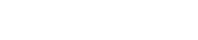高 中1对1课程高三课程

• 院校代码查询

 按高校地区: 全部 上海 云南 内蒙古 北京 吉林 四川 天津 宁夏 安徽 山东 山西 广东 广西 新疆 江苏 江西 河北 河南 浙江 海南 港澳台 湖北 湖南 甘肃 福建 西藏 贵州 辽宁 重庆 陕西 青海 黑龙江 高校类型: 全部 综合类 工科类 师范类 财经类 政法类 语言类 医药类 农业类 林业类 民族类 艺术类 体育类 军事类 高校特色: 全部 双一流 211高校 985高校 研究生 教育部直属 学历层次: 全部 本科 高职专科 独立学院 民办高校 其他

```

```
`	`

```

```
`	`

```

```
`	`

```

```
`	`

```

```
`	`

```

```
`	`

```

```
`	`

```

```
`	`

```

```
`	`

```

```
`	`

```

```
`	`

```

```
`	`

```

```
`	`

```

```
`	`

```

```
`	`

```

```
`	`

```

```
`	`

```

```
`	`

```

```
`	`

```

```
`	`

```

```
`	`

```

```
`	`

```

```
`	`

```

```
`	`

```

```
`	`

```

```
`	`

```

```
`	`

```

```
`	`

```

```
`	`

```

```
`	`

```

```
`	`

```

```
`	`

```

```
`	`

```

```
`	`

```

```
`	`

```

```
`	`

```

```
`	`

```

```
`	`

```

```
`	`

```

```
`	`

```

```
`	`

```

```
`	`

```

```
`	`

```

```
`	`

```

```
`	`

```

```
`	`

```

```
`	`

```

```
`	`

```

```
`	`

```

```
`	`

```

```
`	`

```

```
`	`

```

```
`	`

```

```
`	`

```

```
`	`

```

```
`	`

```

```
`	`

```

```
`	`

```

```
`	`

```

```
`	`

```

```
`	`

```

```
`	`

```

```
`	`

```

```
`	`

```

```
`	`

```

```
`	`

```

```
`	`

```

```
`	`

```

```
`	`

```

```
`	`

```

```
`	`

```

```
`	`

```

```
`	`

```

```
`	`

```

```
`	`

```

```
`	`

```

```
`	`

```

```
`	`

```

```
`	`

```

```
`	`

```

```
`	`

```

```
`	`

```

```
`	`

```

```
`	`

```

```
`	`

```

```
`	`

```

```
`	`

```

```
`	`

```

```
`	`

```

```
`	`

```

```
`	`

```

```
`	`

```

```
`	`

```

```
`	`

```

```
`	`

```

```
`	`

```

```
`	`

```

```
`	`

```

```
`	`

```

```
`	`

```

```
`	`

```

```
`	`

```

```
`	`

```

```
`	`

```

```
`	`

```

```
`	`

```

```
`	`

```

```
`	`

```

```
`	`

```

```
`	`

```

```
`	`

```

```
`	`

```

```
`	`

```

```
`	`

```

```
`	`

```

```
`	`

```

```
`	`

```

```
`	`